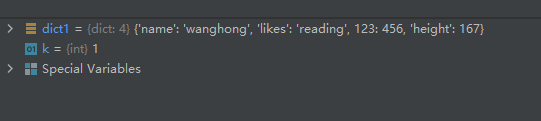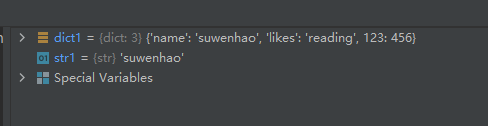# Summary of operation methods of Python Dictionary (dict) (about 18 operation methods), with sample code attached

2022-06-24 04:27:03

Statement ： Blogger ( Haohong image algorithm ) When writing this blog post , With Python Is the version number 3.9.10.

Dictionaries (dict) The format is as follows ：

``````dict1 = {
key1 : value1, key2 : value2, key3 : value3 }
``````

From the above format we can see ：
Each key value of the dictionary key=>value Divide... With a colon , Each pair is separated by commas , The whole dictionary is enclosed in curly brackets {} in .

In addition, the dictionary requires that the key must be unique , But values don't have to be .

# 01- Dictionary creation

``````#  Create a dictionary with content
dict1 = {
'name': 'suwenhao', 'likes': 'reading', 123: 456}

#  Method one creates an empty dictionary
dict2 = {
}

#  Method 2: create an empty dictionary
dict3 = dict()
``````

The operation results are as follows ：## 01- attach 1- Utilization method fromkeys() Quickly generate multi key dictionary with tuples

The grammar is as follows ：

``````dict.fromkeys(keys, value)
``````

keys — It's necessary . Specify the iteratable object for the new dictionary key .
value— Optional . Values of all keys . The default value is None.
The first example code is as follows ：

``````x = ('key1', 'key2', 'key3')
y = 0

dict1 = dict.fromkeys(x, y)
``````

The operation results are as follows ：The second example code ：

``````x = ('key1', 'key2', 'key3')
y = (61, 62, 63)

dict1 = dict.fromkeys(x, y)
``````

The operation results are as follows ：Be careful ：key1 The key value of is not 61, It's a tuple (61, 62, 63), this Pay attention to .

The third example code ：

``````x = ('key1', 'key2', 'key3')
dict1 = dict.fromkeys(x)
``````

The operation results are as follows ：The sample code is as follows ：

``````dict1 = {
'name': 'suwenhao', 'likes': 'reading', 123: 456}

str1 = dict1['name']
value1 = dict1
``````

The operation results are as follows ：# 03- Update the value of a key in the dictionary 、 New key value

The sample code is as follows ：

``````dict1 = {
'name': 'suwenhao', 'likes': 'reading', 123: 456}
k = 1
dict1['name'] = 'wanghong'  #  Update key name Value
dict1['height'] = 167  #  Increase key value
``````

The operation results are as follows ：put questions to ： Why is there a sentence inserted in the middle "k = 1", See the following blog post for the answers ：
https://blog.csdn.net/wenhao_ir/article/details/125416514

# 04- use del Statement to delete a key value from the dictionary

The sample code is as follows ：

``````dict1 = {
'name': 'suwenhao', 'likes': 'reading', 123: 456}
del dict1['name']
``````

The operation results are as follows ：# 05- use del Statement to delete the entire dictionary

The sample code is as follows ：

``````dict1 = {
'name': 'suwenhao', 'likes': 'reading', 123: 456}
dict2 = {
'name': 'wanghong', 'likes': 'sing', 999: 704}
del dict1
``````

The operation results are as follows ：# 06- Method of use clear() Empty dictionary

The sample code is as follows ：

``````dict1 = {
'name': 'suwenhao', 'likes': 'reading', 123: 456}
dict1.clear()
``````

The operation results are as follows ：# 07- Use functions len() Count the number of dictionary key value pairs

The sample code is as follows ：

``````dict1 = {
'name': 'suwenhao', 'likes': 'reading', 123: 456}
len1 = len(dict1)
``````

The operation results are as follows ：# 06- Use functions str() String dictionary

The sample code is as follows ：

``````dict1 = {
'name': 'suwenhao', 'likes': 'reading', 123: 456}
str1 = str(dict1)
print(str1)
``````

The operation results are as follows ：# 07- Usage method copy() Realize deep copy of dictionary

The sample code is as follows ：

``````dict1 = {
'name': 'suwenhao', 'likes': 'reading', 123: 456}
dict2 = dict1.copy()
dict1['name'] = 'zhangsan'
``````From the running results, we can see that , Method copy() It does realize deep copy , change dict1 The key value of does not affect dict2 Value .

# 08- Use “=” Implement a shallow copy of the dictionary

The sample code is as follows ：

``````dict1 = {
'name': 'suwenhao', 'likes': 'reading', 123: 456}
dict2 = dict1
dict1['name'] = 'zhangsan'
``````

The operation results are as follows ：From the above running results, we can see that ,dict1 The modification of key values affects dict2 Key value of , Both of them share memory space .

# 09- Use “in” Determine whether a key is in the dictionary

The sample code is as follows ：

``````dict1 = {
'name': 'suwenhao', 'likes': 'reading', 123: 456}
bool1 = 'name' in dict1
bool2 = 'weight' in dict1
``````

The operation results are as follows ：# 10- Usage method items() View object that gets dictionary keys and values

The sample code is as follows ：

``````dict1 = {
'name': 'suwenhao', 'likes': 'reading', 123: 456}
dict1_items = dict1.items()
print(dict1_items)
``````

The operation results are as follows ：so , This view object is to replace the dictionary object with a formal representation .
For example, the original key value form is ：

``````'name': 'suwenhao'
``````

Convert to view object (dict_items) The following is the form ：

``````('name', 'suwenhao')
``````

It is worth noting that , The original key value must be changed , The corresponding view object will also change , Take an example ：

``````dict1 = {
'name': 'suwenhao', 'likes': 'reading', 123: 456}
dict1_items = dict1.items()
dict1['name'] = 'zhangsan'
print(dict1_items)
``````

The results are shown in the following figure ：View objects can use functions list() Convert to list type , Like the following example ：

``````dict1 = {
'name': 'suwenhao', 'likes': 'reading', 123: 456}
dict1_items = dict1.items()
list1 = list(dict1_items)
``````

The operation results are as follows ：As can be seen from the above results , After converting to a list object , Each key value is a tuple (tuple).

About view objects , Be careful , We can't make any changes to the view object , Because the view objects of the dictionary are read-only .

# 11- Usage method keys() Get the view object of the dictionary key

The sample code is as follows ：

``````dict1 = {
'name': 'suwenhao', 'likes': 'reading', 123: 456}
dict1_keys = dict1.keys()
list1 = list(dict1_keys)
``````

The operation results are as follows ：For relevant knowledge and instructions, please refer to “10- Usage method items() View object that gets dictionary keys and values ”

# 12- Usage method values() View object that gets the value of the dictionary key

The sample code is as follows ：

``````dict1 = {
'name': 'suwenhao', 'likes': 'reading', 123: 456}
dict1_values = dict1.values()
list1 = list(dict1_values)
``````

The operation results are as follows ：For relevant knowledge and instructions, please refer to “10- Usage method items() View object that gets dictionary keys and values ”

# 13-1- Usage method get() Returns the value of a key

The sample code is as follows ：

``````dict1 = {
'name': 'suwenhao', 'likes': 'reading', 123: 456}

str1 = dict1.get('name')
``````

The operation results are as follows ：# 13-2- Usage method setdefault() Returns the value of a key ( If the key doesn't exist , Automatically create )

Method setdefault() The grammar is as follows ：

``````dict.setdefault(key, default=None)
``````

Parameters ：
key – Find the key value .
default – When the key doesn't exist , Set the default key value .
Return value ：
If key stay In the dictionary , Return the corresponding value . If it's not in the dictionary , The insert key And set the default value default, And back to default ,default The default value is None.
The sample code is as follows ：

``````dict1 = {
'name': 'suwenhao', 'likes': 'reading', 123: 456}

str1 = dict1.setdefault('name', 'zhangsan')
str2 = dict1.setdefault('likes', None)
temp1 = dict1.setdefault('weight', None)

``````

The operation results are as follows ：Result analysis ：
Because of the key ’name’ Is there , So the sentence ：

``````str1 = dict1.setdefault('name', 'zhangsan')
``````

Does not change its key value , And returned its key value ‘suwenhao’
Because of the key ’weight’ It doesn't exist , So in the dictionary dict1 New key in ’weight’, Its value is set to None.

# 14- Usage method update() Add the key value of one dictionary to another dictionary

The sample code is as follows ：

``````dict1 = {
'name': 'suwenhao', 'likes': 'reading', 123: 456}
dict2 = {
'weight': 30}

dict1.update(dict2)
``````

The operation results are as follows ：# 15- Usage method pop() Delete a pair of key values , And returns the value of the key

The sample code is as follows ：

``````dict1 = {
'name': 'suwenhao', 'likes': 'reading', 123: 456}

temp1 = dict1.pop('name')
``````

The operation results are as follows ：# 16- Usage method popitem() Delete the last inserted key value , And returns the key and its value

The last key value inserted is usually the last key value .
The sample code is as follows ：

``````dict1 = {
'name': 'suwenhao', 'likes': 'reading', 123: 456}

temp1 = dict1.popitem()
``````

The operation results are as follows ：# 17- There are two points to pay attention to when using the key

1、 The same key is not allowed to appear twice . When creating, if the same key is assigned twice , The latter value will be remembered , The following example ：

``````dict1 = {
'name': 'suwenhao', 'likes': 'reading', 123: 456, 'name': 'zhangsan'}
str1 = dict1['name']
``````

The operation results are as follows ：2、 The key must be immutable , So you can use numbers , A string or tuple acts as , Not with lists , As the following statement ：

``````dict1 = {
['name']: 'suwenhao', 'likes': 'reading', 123: 456}
``````

When running, the error is as follows ：Reference material ：
https://blog.csdn.net/wenhao_ir/article/details/125100220